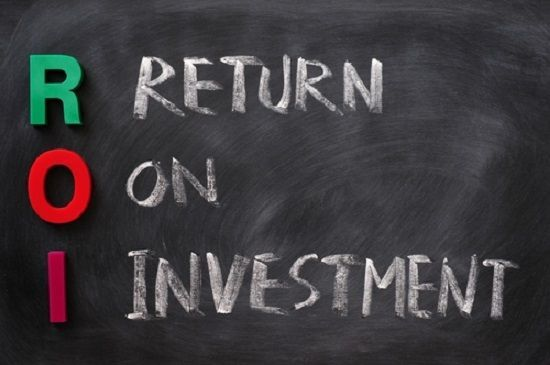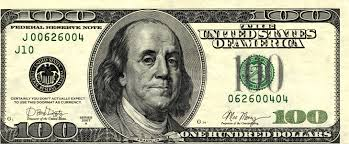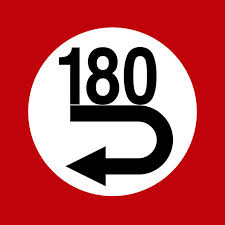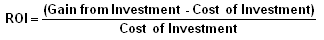What is ROI (Return on Investment)?

ROI is basically a term that help us understand.

How much money we earned from an investment.

For example:

We invest \$100 in a cfd positionWe won a contract and earned \$80Overall, we got \$180 back while investing \$100The formula to calculate ROI isSo in our case ROI is (180-100)/100 = 80%There is more to understand ROI, but this is all you need to know for the basics.